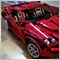Draw_Line: does not draw the buffer (MQL5)768

I am creating an indicator that draws a buffer line on the moving average values but the buffer is not written to the graph.

#property indicator_chart_window
#property indicator_buffers 1
#property indicator_plots   1
#property indicator_label1  "Line"
#property indicator_type1   DRAW_LINE
#property indicator_color1  clrBlue
#property indicator_style1  STYLE_SOLID
#property indicator_width1  2

double LineBuffer[];
int periodi=21;

int OnInit()
{

SetIndexBuffer(0,LineBuffer,INDICATOR_DATA);

return(INIT_SUCCEEDED);
}

double calculateoMedia(int begin){

double media = 0;

for(int n=begin+periodi-1;n>=begin;n--){

media += iClose(Symbol(),0,n);
}
media /= periodi;

return media;
}

int OnCalculate(const int rates_total,
const int prev_calculated,
const datetime &time[],
const double &open[],
const double &high[],
const double &low[],
const double &close[],
const long &tick_volume[],
const long &volume[],

for(int i=110; i>=0; i--){

if(i < 89){

LineBuffer[i]=calculateoMedia(i);
}
}

return(rates_total);
}

instead if I use the data of the High Value Bars, the buffer is written without problems

for(int i=0;i<rates_total;i++)
{
LineBuffer[i]=high[i];
}605

this function :

double calculateoMedia(int begin){

double media = 0;

for(int n=begin+periodi-1;n>=begin;n--){

media += iClose(Symbol(),0,n);
}
media /= periodi;

return media;
}

is it not  same as :

double media = iMA(Symbol(),0,periodi,0,MODE_SMA,PRICE_CLOSE,begin);605

if yes did you test :

for(int i=88; i>=0; i--) LineBuffer[i]=iMA(_Symbol,_Period,periodi,0,MODE_SMA,PRICE_CLOSE,i);768

I'm writing in mql5 and my function calculates the moving average and returns the value to be attributed to the Buffer and is correct because in mql4 it works regularly but I don't understand why in mql5 no.26790

1. paul selvan:

this function :

is it not  same as :

They are exactly the same. That is how MT4's iMA does it.

2. fly7680 in mql4 it works regularly but I don't understand why in mql5 no.
Your code looks perfect. Is there a problem with LineBuffer being a buffer?1876

fly7680:

I am creating an indicator that draws a buffer line on the moving average values but the buffer is not written to the graph.

instead if I use the data of the High Value Bars, the buffer is written without problems

You'll need two more lines in your OnInit() function, as follows:

// So that LineBuffer refers to the latest, rather than the earliest, bar.
ArraySetAsSeries(LineBuffer,true);

// With the above set, the left end of the line drops vertically to zero,
// so you need this to indicate that you don't want zero values to be drawn.
PlotIndexSetDouble(0,PLOT_EMPTY_VALUE,0);